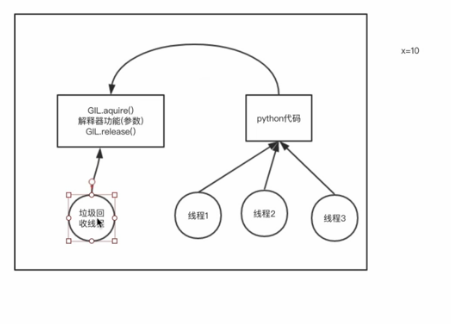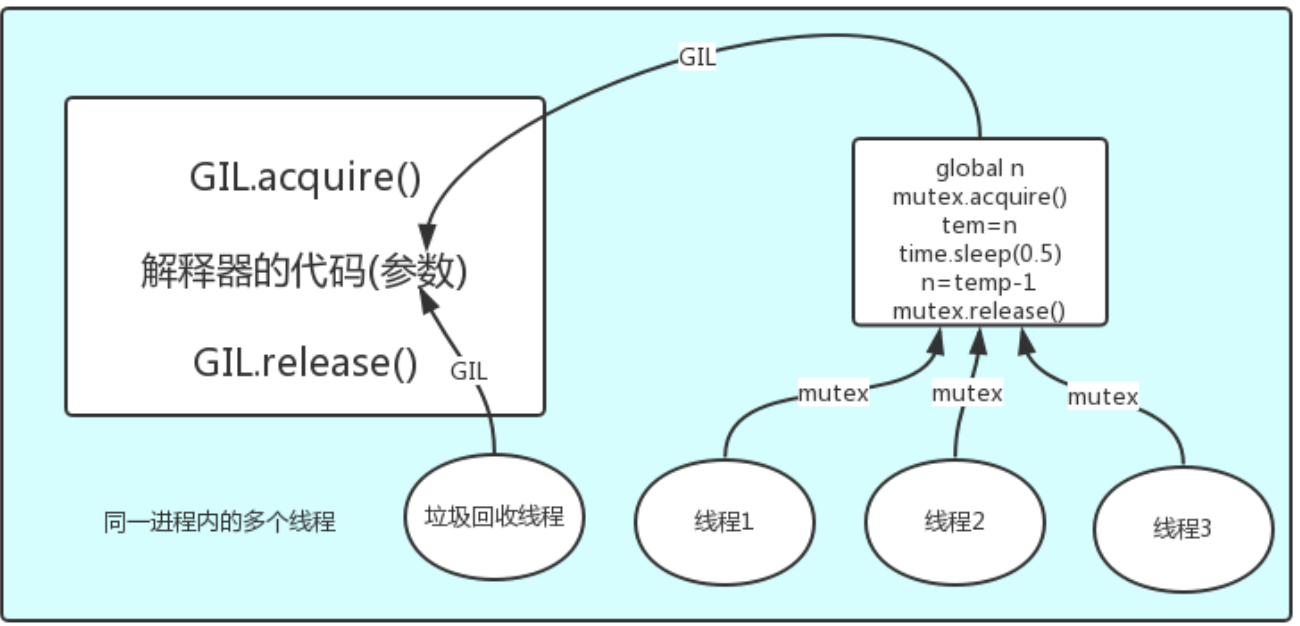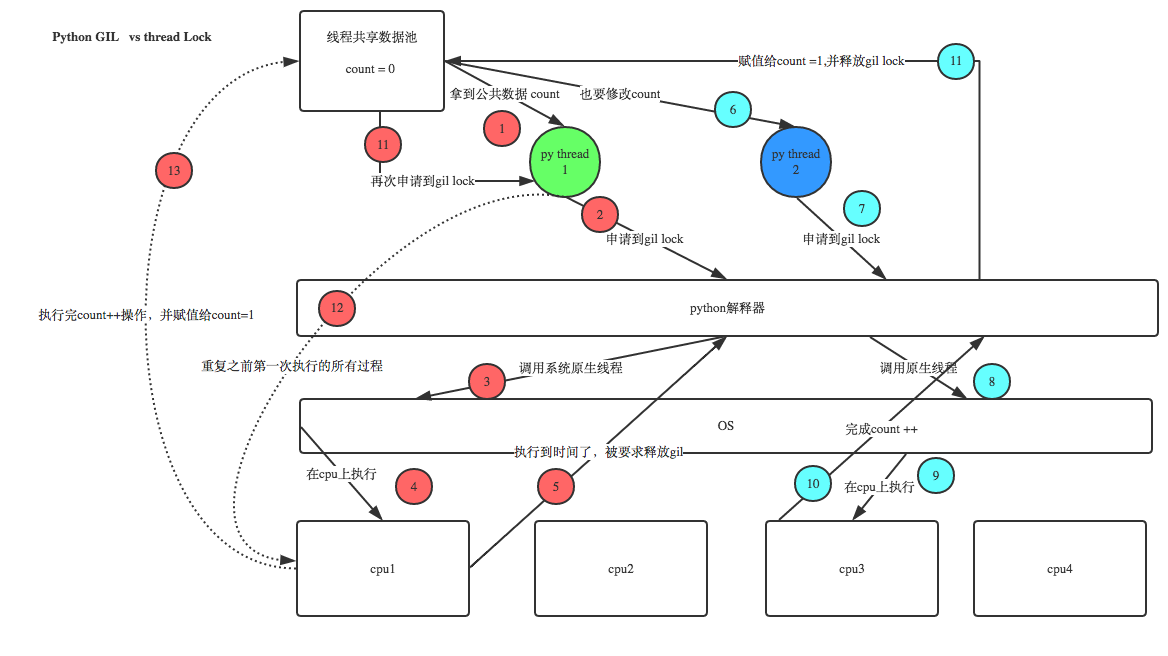# 线程

## 开启线程的两种方式

```# import time
# import random
# from threading import Thread
#
# def piao(name):
#     print('%s piaoing' %name)
#     time.sleep(random.randrange(1,5))
#     print('%s piao end' %name)
#
# if __name__ == '__main__':
#     t1=Thread(target=piao,args=('egon',))
#     t1.start()
#     print('主线程')
#只要是开了个进程，只是开了个内存空间，其实它会自动创建个线程。上边一共有2个线程；

import time
import random
from threading import Thread

class MyThread(Thread):
def __init__(self,name):
super().__init__()
self.name=name

def run(self):
print('%s piaoing' %self.name)

time.sleep(random.randrange(1,5))
print('%s piao end' %self.name)
if __name__ == '__main__':
t1=MyThread('egon')
t1.start()
print('主')

egon piaoing

egon piao end```
```#1开进程的开销远大于开线程
import time
from threading import Thread
from multiprocessing import Process
def piao(name):
print("%s piaoing"%name)
time.sleep(2)
print("%s piao end"%name)
if __name__ == "__main__":
#p1 = Process(target=piao, args=('egon', )) #它要申请内存空间
#p1.start()
t1 = Thread(target=piao, args=('egon', ))
t1.start()#信号发出以后，线程立马就起来了
print('主线程')```
```#2同一个进程内多个线程共享该进程的地址空间
from threading import Thread
from multiprocessing import Process
n = 100
def task():
global n
n = 0
if __name__ == "__main__":
# p1 = Process(target=task, ) #它要申请内存空间
# p1.start() #开一个子进程，会copy主进程的内存空间
# p1.join()#确保它执行完了  打印出的是100

t1 = Thread(target=task, )
t1.start()#共享
t1.join()
print('主线程', n) #子进程改了，不影响主进程，改的是它自己内存空间的，打印的是0```
```# 3、瞅一眼pid
from threading import Thread
from multiprocessing import Process,current_process
import os
def task():
# print(current_process().pid)  #查看线程id，不能看父进程（用os)的
print('子进程PID:%s  父进程的PID:%s' %(os.getpid(),os.getppid()))

if __name__ == '__main__':
p1=Process(target=task,)
p1.start()
# print('主进程',current_process().pid)
print('主进程',os.getpid())

```from threading import Thread
import os
def task():
print('子线程:%s' %(os.getpid())) #一个进程内的线程大家的地位是一样的
if __name__ == '__main__':
t1=Thread(target=task,)
t1.start()
print('主线程',os.getpid()) #这两个线程的同属于一个进程

## Thread对象的其他属性或方法

``````Thread实例对象的方法
# isAlive(): 返回线程是否活动的。
# getName(): 返回线程名。
# setName(): 设置线程名。

threading模块提供的一些方法：
# threading.currentThread(): 返回当前的线程变量。
# threading.enumerate(): 返回一个包含正在运行的线程的list。正在运行指线程启动后、结束前，不包括启动前和终止后的线程。
# threading.activeCount(): 返回正在运行的线程数量，与len(threading.enumerate())有相同的结果。``````
```from threading import Thread,currentThread,active_count,enumerate
import time
def task():
print('%s is ruuning' %currentThread().getName())
time.sleep(2)
print('%s is done' %currentThread().getName())
if __name__ == '__main__':
t=Thread(target=task,name='子线程1')
t.start()
# t.setName('儿子线程1') #设置名字
# t.join()
# print(t.getName()) #t就是currentThread()
# currentThread().setName('主线程')
# print(t.isAlive())  #查看线程是否还活着，加了t.join就死掉了

# print('主线程',currentThread().getName())  看下主线程用那个currentThread，查看当前线程名

# t.join()
# print(active_count()) #活跃的线程数 只剩下主线程了，因为你join了
print(enumerate())  #把当前活跃的线程对象拿过来```

## 守护线程

```1、对主进程来说，运行完毕指的是主进程代码运行完毕

2、对主线程来说，运行完毕指的是主线程所在的进程内所有非守护线程统统运行完毕，主线程才算运行完毕

1.1、主进程在其代码结束后就已经算运行完毕了（守护进程在此时就被回收）,然后主进程会一直等非守护的子进程都运行完毕后回收子进程的资源(否则会产生僵尸进程)，才会结束，

2.1、主线程在其他非守护线程运行完毕后才算运行完毕（守护线程在此时就被回收）。因为主线程的结束意味着进程的结束，进程整体的资源都将被回收，而进程必须保证非守护线程都运行完毕后才能结束。```
```from threading import Thread
import time

def sayhi(name):
time.sleep(2)
print('%s say hello' %name)

if __name__ == '__main__':
t=Thread(target=sayhi,args=('egon',))
# t.setDaemon(True) #必须在t.start()之前设置；两种守护线程设置方式，另外一种是下面
t.daemon=True
t.start() #造线程，立马就造出来了，睡2s就足够打印下面"主线程'了

print('主线程') #2s把主线程都运行完了；主线程没有要等的了，然后就死掉了，守护进程跟着死，就不会打印上边那个say hello
print(t.is_alive()) #打印这2s也足够它运行了

True```
```from threading import Thread
import time
def foo():
print(123)
time.sleep(1)
print("end123")
def bar():
print(456)
time.sleep(3) #t2非守护进程要等待3s，睡1s开到“end123”，再睡2s看到“end456”
print("end456")
if __name__ == '__main__':
t1=Thread(target=foo)
t2=Thread(target=bar)
#一共3个线程；t1为守护线程，t2为非守护线程；主线程运行完就盯着非守护进程运行完，主线程才运行完
t1.daemon=True
t1.start()
t2.start()
print("main-------")

123
456
main-------
end123
end456```

## 互斥锁

```#mutex
from threading import Thread,Lock
import time
n = 100
def task():
global n
mutex.acquire() #1个线程起来先去抢一把锁，在它睡0.1s时，其他99个线程同时会去抢锁，等第一个执行完后抢得锁，这时候n=99，然后再来回循环
temp = n
time.sleep(0.1) #睡0.1s就足够其他99个线程启动运行了；都停在这睡之前都拿到了n=100了
n = temp - 1  #这100个数据都改成了99，数据变得不安全了，得加把锁
mutex.release()
if __name__ == '__main__':
mutex = Lock()
t_l = []
for i in range(100):
t = Thread(target=task) #在1个进程里边开了100个线程，共享空间的
t_l.append(t)
t.start()
for t in t_l:
t.join()
print('主', n)

## GIL的基本概念

同样一段代码可以通过CPython，PyPy，Psyco等不同的Python执行环境来执行。像其中的JPython就没有GIL。然而因为CPython是大部分环境下默认的Python执行环境。所以在很多人的概念里CPython就是Python，也就想当然的把GIL归结为Python语言的缺陷。所以这里要先明确一点：GIL并不是Python的特性，Python完全可以不依赖于GIL。

``在Cpython解释器中，同一个进程下开启的多线程，同一时刻只能有一个线程执行，无法利用多核优势``

GIL本质就是一把互斥锁，既然是互斥锁，所有互斥锁的本质都一样，都是将并发运行变成串行，以此来控制同一时间内共享数据只能被一个任务所修改，进而保证数据安全。可以肯定的一点是：保护不同的数据的安全，就应该加不同的锁。```############验证python test.py只会产生一个进程
#test.py内容
import os,time
print(os.getpid())
time.sleep(1000)

#打开终端执行
python3 test.py

#在windows下查看
tasklist |findstr python

#在linux下下查看
ps aux |grep python```

```1、所有数据都是共享的，这其中，代码作为一种数据也是被所有线程共享的（test.py的所有代码以及Cpython解释器的所有代码）

2、所有线程的任务，都需要将任务的代码当做参数传给解释器的代码去执行，即所有的线程要想运行自己的任务，首先需要解决的是能够访问到解释器的代码。```GIL与自定义互斥锁

GIL保护的是解释器级别跟垃圾回收机制有关的数据；

mutex保护的是自己的数据；```1、100个线程去抢GIL锁，即抢执行权限
2、肯定有一个线程先抢到GIL（暂且称为线程1），然后开始执行，一旦执行就会拿到lock.acquire()
3、极有可能线程1还未运行完毕，就有另外一个线程2抢到GIL，然后开始运行，但线程2发现互斥锁lock还未被线程1释放，于是阻塞，被迫交出执行权限，即释放GIL
4、直到线程1重新抢到GIL，开始从上次暂停的位置继续执行，直到正常释放互斥锁lock，然后其他的线程再重复2 3 4的过程```

GIL与多线程

有了GIL的存在，同一时刻同一个进程内的多个线程，只能有一个出来执行；

进程可以利用多核，但是开销大，而python的多线程开销小，但却无法利用多核优势，

```1、cpu到底是用来做计算的，还是用来做I/O的？

2、多cpu，意味着可以有多个核并行完成计算，所以多核提升的是计算性能

3、每个cpu一旦遇到I/O阻塞，仍然需要等待，所以多核对I/O操作没什么用处```
```1、对计算来说，cpu越多越好，但是对于I/O来说，再多的cpu也没用
2、当然对运行一个程序来说，随着cpu的增多执行效率肯定会有所提高（不管提高幅度多大，总会有所提高），这是因为一个程序基本上不会是纯计算或者纯I/O，所以我们只能相对的去看一个程序到底是计算密集型还是I/O密集型，从而进一步分析python的多线程到底有无用武之地```

``````方案一：开启四个进程

``````

``````如果四个任务是计算密集型，没有多核来并行计算，方案一徒增了创建进程的开销，方案二胜

``````

``````如果四个任务是计算密集型，多核意味着并行计算，在python中一个进程中同一时刻只有一个线程执行用不上多核，方案一胜

``````

```##计算密集型，应该用多进程
from multiprocessing import Process
from threading import Thread
import os,time
def work():
res=0
for i in range(100000000):
res*=i

if __name__ == '__main__':
l=[]
print(os.cpu_count()) #本机为4核
start=time.time()
for i in range(4):
p=Process(target=work) #多进程 耗时5s多 牺牲开进程的开销用上了多核优势
#p=Thread(target=work) #多线程 耗时18s多
l.append(p)
p.start()
for p in l:
p.join()
stop=time.time()
print('run time is %s' %(stop-start))```
```#I/O密集型用多线程
from multiprocessing import Process
from threading import Thread
import threading
import os,time
def work():
time.sleep(2)
#print('===>')
if __name__ == '__main__':
l=[]
print(os.cpu_count()) #本机为4核
start=time.time()
for i in range(400):
#p=Process(target=work) #耗时12s多,大部分时间耗费在创建进程上
p=Thread(target=work) #耗时2s多 ，消耗的就是来回切的时间
l.append(p)
p.start()
for p in l:
p.join()
stop=time.time()
print('run time is %s' %(stop-start))```
``````多线程用于IO密集型，如socket，爬虫，web

## 死锁与递归锁

```# 死锁
from threading import Thread,Lock
import time
mutexA=Lock()
mutexB=Lock()

class MyThread(Thread):
def run(self):
self.f1()
self.f2()
def f1(self):
mutexA.acquire()
print('%s 拿到了A锁' %self.name)

mutexB.acquire()  #线程1拿到了B锁，还没人跟它抢，最多其他线程都去抢A锁
print('%s 拿到了B锁' %self.name)
mutexB.release()

mutexA.release()

def f2(self):
mutexB.acquire()
print('%s 拿到了B锁' % self.name)
time.sleep(0.1)

mutexA.acquire()  #线程1执行f2，拿到B锁后，又去拿A锁，但这个时候A锁在第二个进程里边拿着呢
print('%s 拿到了A锁' % self.name)
mutexA.release()

mutexB.release()

if __name__ == '__main__':
for i in range(10):
t=MyThread()
t.start()

Thread-1 拿到了A锁
Thread-1 拿到了B锁
Thread-1 拿到了B锁
Thread-2 拿到了A锁```
```# 互斥锁只能acquire一次
# from threading import Thread,Lock
#
# mutexA=Lock()
#
# mutexA.acquire()
# mutexA.release()```

```# 递归锁:可以连续acquire多次，每acquire一次计数器+1，只有计数为0时，其他线程才能被抢到acquire
from threading import Thread,RLock
import time

mutexB=mutexA=RLock()

class MyThread(Thread):
def run(self):
self.f1()
self.f2()

def f1(self):
mutexA.acquire()
print('%s 拿到了A锁' %self.name)

mutexB.acquire()  #这个时候计数器为2，其他进程都不能跟它抢
print('%s 拿到了B锁' %self.name)
mutexB.release()

mutexA.release()
def f2(self):
mutexB.acquire()
print('%s 拿到了B锁' % self.name)
time.sleep(7)

mutexA.acquire()
print('%s 拿到了A锁' % self.name)
mutexA.release()

mutexB.release()
if __name__ == '__main__':
for i in range(10):
t=MyThread()
t.start()

Thread-1 拿到了A锁
Thread-1 拿到了B锁
Thread-1 拿到了B锁
Thread-1 拿到了A锁
Thread-2 拿到了A锁
Thread-2 拿到了B锁
Thread-2 拿到了B锁
Thread-2 拿到了A锁
Thread-4 拿到了A锁
Thread-4 拿到了B锁
Thread-4 拿到了B锁
Thread-4 拿到了A锁
Thread-6 拿到了A锁
Thread-6 拿到了B锁
Thread-6 拿到了B锁
Thread-6 拿到了A锁
Thread-8 拿到了A锁
Thread-8 拿到了B锁
Thread-8 拿到了B锁
Thread-8 拿到了A锁
Thread-10 拿到了A锁
Thread-10 拿到了B锁
Thread-10 拿到了B锁
Thread-10 拿到了A锁
Thread-5 拿到了A锁
Thread-5 拿到了B锁
Thread-5 拿到了B锁
Thread-5 拿到了A锁
Thread-9 拿到了A锁
Thread-9 拿到了B锁
Thread-9 拿到了B锁
Thread-9 拿到了A锁
Thread-7 拿到了A锁
Thread-7 拿到了B锁
Thread-7 拿到了B锁
Thread-7 拿到了A锁
Thread-3 拿到了A锁
Thread-3 拿到了B锁
Thread-3 拿到了B锁
Thread-3 拿到了A锁```

## 信号亮

信号量也是一把锁，可以指定信号量为5，对比互斥锁同一时间只能有一个任务抢到锁去执行，信号量同一时间可以有5个任务拿到锁去执行，如果说互斥锁是合租房屋的人去抢一个厕所，那么信号量就相当于一群路人争抢公共厕所，公共厕所有多个坑位，这意味着同一时间可以有多个人上公共厕所，但公共厕所容纳的人数是一定的，这便是信号量的大小。

```from threading import Thread, Semaphore, currentThread
import time, random
sm = Semaphore(3) #有3个人可以抢到
def task():
# sm.acquire()
# print('%s in'%currentThread().getName())
# sm.release()
#加锁也可以用一个上下文管理的方式如下
with sm:
print('%s in'%currentThread().getName())
time.sleep(random.randint(1,3))
if __name__ == '__main__':
for i in range(10):
t = Thread(target = task)
t.start()

Thread-1 in
Thread-2 in
Thread-3 in
Thread-4 in
Thread-5 in
Thread-6 in
Thread-7 in
Thread-8 in
Thread-9 in
Thread-10 in```

## Event事件

线程的一个关键特性是每个线程都是独立运行且状态不可预测。如果程序中的其他线程需要通过判断某个线程的状态来确定自己下一步的操作,这时线程同步问题就会变得非常棘手。为了解决这些问题,我们需要使用threading库中的Event对象。 对象包含一个可由线程设置的信号标志,它允许线程等待某些事件的发生。在 初始情况下,Event对象中的信号标志被设置为假。如果有线程等待一个Event对象, 而这个Event对象的标志为假,那么这个线程将会被一直阻塞直至该标志为真。一个线程如果将一个Event对象的信号标志设置为真,它将唤醒所有等待这个Event对象的线程。如果一个线程等待一个已经被设置为真的Event对象,那么它将忽略这个事件, 继续执行。

```from threading import Event

event.isSet()：返回event的状态值；

event.wait()：如果 event.isSet()==False将阻塞线程；

event.set()： 设置event的状态值为True，所有阻塞池的线程激活进入就绪状态， 等待操作系统调度；

event.clear()：恢复event的状态值为False。```
```#应用场景

from threading import Thread, Event
import time
event = Event() ###event.wait() #一直在那等着；直到等到event.set()才结束了
def student(name):
print('学生%s 正在听课 '%name)
event.wait(2) #可以设置个超时时间，过了3s即使没有给我发set信号我也可以接着干其他的
print('学生%s 课间活动 '%name)
def teacher(name):
print('老师%s 正在授课 '%name)
time.sleep(8)
event.set()
if __name__ == '__main__':
stu1 = Thread(target=student, args=('kris', ))
stu2 = Thread(target=student, args=('alex', ))
stu3 = Thread(target=student, args=('alen', ))
t1=Thread(target=teacher,args=('egon',))

stu1.start()
stu2.start()
stu3.start()
t1.start()

```from threading import Thread,Event,currentThread
import time
event=Event()

def conn(): #尝试链接，检测是否链接成功；event事件，一个等，一个唤醒
n=0
while not event.is_set(): #循环的发请求
if n == 3:
print('%s try too many times' %currentThread().getName())
return
print('%s try %s' %(currentThread().getName(),n))
event.wait(0.5) #等5s时间尝试，
n+=1

print('%s is connected' %currentThread().getName())

def check(): #检测服务端是否正常运行
print('%s is checking' %currentThread().getName())
time.sleep(5)
event.set()

if __name__ == '__main__':
for i in range(3):
t=Thread(target=conn)
t.start()
t=Thread(target=check)
t.start()

Thread-1 try 0
Thread-2 try 0
Thread-3 try 0
Thread-4 is checking
Thread-2 try 1
Thread-3 try 1
Thread-1 try 1
Thread-1 try 2
Thread-2 try 2
Thread-3 try 2
Thread-2 try too many times
Thread-3 try too many times
Thread-1 try too many times```

## 定时器

```from threading import Timer

def task(name):
print('hello %s' %name)
t=Timer(5,task,args=('egon',))
t.start()

hello egon```
```from threading import Timer
import random
class Code:
def __init__(self):
self.make_cache()  #最开始实例化的时候就先拿到一个验证码；

def make_cache(self, interval=9): #做缓存功能
self.cache = self.make_code()
print(self.cache)
self.t = Timer(interval, self.make_cache )
self.t.start()
def make_code(self, n=4): #一个随机字符串
res = ''
for i in range(n):
s1 = str(random.randint(0, 9))#转成str为了拼接字符串
s2 = chr(random.randint(65, 90)) #拿到字母，对应ascii表里边的
res += random.choice([s1, s2])
return res
def check(self):
while True:
code = input('请输入你的验证码>>>:').strip() #你输不对我就隔5s刷新一下
if code.upper() == self.cache:
print('验证码输入正确')
self.t.cancel()
break
obj = Code()
obj.check()```

## 线程queue

```###基本用法

import queue
q=queue.Queue(3) #先进先出->队列

q.put('first')
q.put(2)
q.put('third')
# q.put(4)
# q.put(4,block=False) #等同于 q.put_nowait(4) #不等待
# q.put(4,block=True,timeout=3) #默认为block=True；等3s

print(q.get())
print(q.get())
print(q.get())
# print(q.get(block=False)) #q.get_nowait()
# print(q.get_nowait())

# print(q.get(block=True,timeout=3))```
```##跟上边用法一样
import queue
q=queue.LifoQueue(3) #后进先出->堆栈
q.put('first')
q.put(2)
q.put('third')

print(q.get())
print(q.get())
print(q.get())

third
2
first```
```import queue
q=queue.PriorityQueue(3) #优先级队列 数字越小优先级越高

q.put((10,'one'))
q.put((40,'two'))
q.put((30,'three'))

print(q.get())
print(q.get())
print(q.get())

(10, 'one')
(30, 'three')
(40, 'two')```

## 多线程实现并发的套接字通信

```#基于线程池实现
##控制了最大并发数，保证了服务端不会无限制的去开启线程
##服务端
from socket import *
from concurrent.futures import ThreadPoolExecutor
def communicate(conn):
while True:
try:
data=conn.recv(1024)
if not data:break
conn.send(data.upper())
except ConnectionResetError:
break
conn.close()

def server(ip,port):
server = socket(AF_INET, SOCK_STREAM)
server.bind((ip,port))
server.listen(5)

while True:
conn, addr = server.accept()
pool.submit(communicate,conn)
server.close()

if __name__ == '__main__':
pool=ThreadPoolExecutor(2) #最多开启2个线程，那么客户端也就最多开启2个了
server('127.0.0.1', 8081)```
```#服务端
from socket import *
from threading import Thread

def communicate(conn):  #通信
while True:
try:
data=conn.recv(1024)
if not data:break
conn.send(data.upper())
except ConnectionResetError:
break
conn.close()
def server(ip,port):  #负责建链接
server = socket(AF_INET, SOCK_STREAM)
server.bind((ip,port))
server.listen(5)
while True:
conn, addr = server.accept() #建链接，建成功一次；立马起个线程；建好链接就给你干通信的活
t=Thread(target=communicate,args=(conn,)) #就像专门聘请个服务员来服务你
t.start()
server.close()

if __name__ == '__main__':
server('127.0.0.1', 8081) #主线程，相当于招待人员```
```#客户端

from socket import *

client=socket(AF_INET,SOCK_STREAM)
client.connect(('127.0.0.1',8081))
while True:
msg=input('>>: ').strip()
if not msg:continue
client.send(msg.encode('utf-8'))
data=client.recv(1024)
print(data.decode('utf-8'))

client.close()```

## 进程池线程池

```from concurrent.futures import ProcessPoolExecutor, ThreadPoolExecutor
#计算密集型多核优势的情况下应该用进程；I/O密集型的应该用线程
import os,time,random
def task(name):
print('name:%s pid:%s run' %(name,os.getpid()))
time.sleep(random.randint(1,3))

if __name__ == '__main__':
pool=ProcessPoolExecutor(4) #先把池子造好，指定最多放4个进程；不指定就是cpu的核字
#pool=ThreadPoolExecutor(5) #在一个进程里边PID是一样的

for i in range(10):
pool.submit(task,'egon%s' %i) #把这10个任务全部丢给池子，没有阻塞
#异步调用，提交完任务不用等着任务执行拿到结果，只负责提交完了立马走
pool.shutdown(wait=True) #执行join操作；默认值就是True #等任务提交结束，把入口关了
print('主')```
```##线程池

from concurrent.futures import ProcessPoolExecutor,ThreadPoolExecutor
from threading import currentThread
import os,time,random

def task():
print('name:%s pid:%s run' %(currentThread().getName(),os.getpid()))
time.sleep(random.randint(1,3))

if __name__ == '__main__':
pool=ThreadPoolExecutor(5)

for i in range(10):
pool.submit(task,)
pool.shutdown(wait=True)
print('主')```

## 异步调用和回调机制

可以为进程池或线程池内的每个进程或线程绑定一个函数，该函数在进程或线程的任务执行完毕后自动触发，并接收任务的返回值当作参数，该函数称为回调函数

```#1、同步调用:提交完任务后，就在原地等待任务执行完毕，拿到结果，再执行下一行代码,导致程序是串行执行
from concurrent.futures import ThreadPoolExecutor
import time
import random

def la(name):
print('%s is laing' %name)
time.sleep(random.randint(3,5))
res=random.randint(7,13)*'#'
return {'name':name,'res':res}

def weigh(shit):
name=shit['name']
size=len(shit['res'])
print('%s 拉了 《%s》kg' %(name,size))

if __name__ == '__main__':
pool=ThreadPoolExecutor(13)

shit1=pool.submit(la,'alex').result() #往池子里提交任务，拿到结果
weigh(shit1) #称重

shit2=pool.submit(la,'wupeiqi').result()
weigh(shit2)

shit3=pool.submit(la,'yuanhao').result()
weigh(shit3)

alex is laing
alex 拉了 《13》kg
wupeiqi is laing
wupeiqi 拉了 《13》kg
yuanhao is laing
yuanhao 拉了 《10》kg```
```#2、异步调用：提交完任务后，不地等待任务执行完毕，
from concurrent.futures import ThreadPoolExecutor
import time
import random

def la(name):
print('%s is laing' %name)
time.sleep(random.randint(3,5))
res=random.randint(7,13)*'#'
return {'name':name,'res':res}

def weigh(shit):
shit=shit.result()
name=shit['name']
size=len(shit['res'])
print('%s 拉了 《%s》kg' %(name,size))

if __name__ == '__main__':
pool=ThreadPoolExecutor(13)

pool.submit(la,'alex').add_done_callback(weigh) #绑定一个回调函数

pool.submit(la,'wupeiqi').add_done_callback(weigh)

pool.submit(la,'yuanhao').add_done_callback(weigh)

alex is laing
wupeiqi is laing
yuanhao is laing
alex 拉了 《11》kg
wupeiqi 拉了 《7》kg
yuanhao 拉了 《8》kg```

```# import requests
# response = requests.get('http://www.cnblogs.com/linhaifeng') #去目标站点下载个文件
# print(response.text)

from concurrent.futures import ThreadPoolExecutor
import requests
import time

def get(url):
print('GET %s' %url)
response=requests.get(url)
time.sleep(3)
return {'url':url,'content':response.text}

def parse(res):  #并发下载，谁下载好了谁就去触发解析功能
res=res.result()
print('%s parse res is %s' %(res['url'],len(res['content'])))

if __name__ == '__main__':
urls=[
'http://www.cnblogs.com/linhaifeng',
'https://www.python.org',
'https://www.openstack.org',
]

pool=ThreadPoolExecutor(2) #线程池，I/O密集型

for url in urls:
pool.submit(get,url).add_done_callback(parse) #异步提交；回调函数

GET http://www.cnblogs.com/linhaifeng
GET https://www.python.org
http://www.cnblogs.com/linhaifeng parse res is 16320
GET https://www.openstack.org
https://www.python.org parse res is 49273
https://www.openstack.org parse res is 64050```